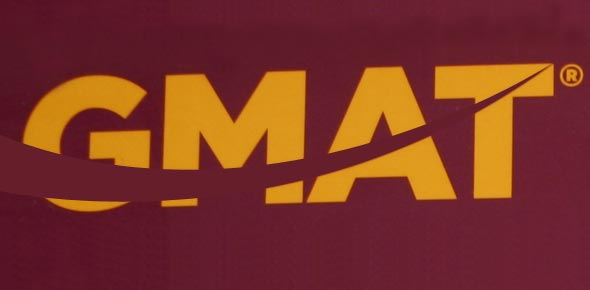# GMATquestions.Org - Mini GMAT Math Test - 10 Questions

10 Questions | Total Attempts: 54SettingsThis GMAT quiz will test your understanding of basic concepts in Number Properties, Inequalities within Data Sufficiency, and Rates on the GMAT. It is a timed test. You have 20 minutes to do 10 questions.

• 1.
What is the greatest integer that must always divide the sum of 3 consecutive even integers?
• A.

2

• B.

3

• C.

6

• D.

12

• E.

15

• 2.
If n is the product of the integers from 1 to 8 inclusive and if p q r and s are positive integers such that  then p+q+r+s=
• A.

3

• B.

7

• C.

8

• D.

11

• E.

12

• 3.
Pierre is driving 60 miles per hour for the first 40 miles of a 80-mile trip. What must be his average speed in the remaining 40 miles in order for his total average speed to be 70 miles per hour?
• A.

70

• B.

75

• C.

80

• D.

84

• E.

85

• 4.
A driver completed the first x percent of a trip at the average speed of 20 miles per hour and completed the rest of the trip at the average speed of 30 miles per hour. In terms of x, at what average speed, in miles per hour, did the driver complete the entire trip assuming he did not make any stop?
• A.
• B.
• C.
• D.
• E.
• 5.
Chris and James are cycling in the same direction, on the same course. At 3:00 pm., Chris, who is peddling at 20 miles per hour, crosses a bridge. One hour later, James passes the same bridge. James is traveling at 24 miles per hour. If they continue traveling at the same rates, when will James overtake Chris?
• A.

9:30 pm

• B.

9:00 pm

• C.

9:45 pm

• D.

10:00 pm

• E.

8:00 pm

• 6.
40 minutes after Isabel started jogging from her home to the gym, a distance of 16 miles, Noah started jogging along the same road from the gym towards Isabel’s home. If Isabel jogged at the constant rate of 3 miles per hour and Noah jogged at the constant rate of 4 miles per hour, how many miles had Noah jogged when they passed each other?
• A.

7

• B.

8

• C.

10

• D.

12

• E.

15

• 7.
Is
• A.

Statement (1) ALONE is sufficient, but statement (2) alone is not sufficient.

• B.

Statement (2) ALONE is sufficient, but statement (1) alone is not sufficient.

• C.

BOTH statements TOGETHER are sufficient, but NEITHER statement ALONE is sufficient.

• D.

EACH statement ALONE is sufficient.

• E.

Statements (1) and (2) TOGETHER are NOT sufficient.

• 8.
What is the value of the integer a?
• A.

Statement (1) ALONE is sufficient, but statement (2) alone is not sufficient.

• B.

Statement (2) ALONE is sufficient, but statement (1) alone is not sufficient.

• C.

BOTH statements TOGETHER are sufficient, but NEITHER statement ALONE is sufficient.

• D.

EACH statement ALONE is sufficient.

• E.

Statements (1) and (2) TOGETHER are NOT sufficient.

• 9.
Is  .
• A.

Statement (1) ALONE is sufficient, but statement (2) alone is not sufficient.

• B.

Statement (2) ALONE is sufficient, but statement (1) alone is not sufficient.

• C.

BOTH statements TOGETHER are sufficient, but NEITHER statement ALONE is sufficient.

• D.

EACH statement ALONE is sufficient.

• E.

Statements (1) and (2) TOGETHER are NOT sufficient.

• 10.
• A.

Statement (1) ALONE is sufficient, but statement (2) alone is not sufficient.

• B.

Statement (2) ALONE is sufficient, but statement (1) alone is not sufficient.

• C.

BOTH statements TOGETHER are sufficient, but NEITHER statement ALONE is sufficient.

• D.

EACH statement ALONE is sufficient.

• E.

Statements (1) and (2) TOGETHER are NOT sufficient.

Related TopicsBack to top